# 33 Expressions and Their Structure

 33 Expressions and Their Structure
We’ve now seen all sorts of things that exist in the Wolfram Language: lists, graphics, pure functions and much more. And now we’re ready to discuss a very fundamental fact about the Wolfram Language: that each of these thingsand in fact everything the language deals withis ultimately constructed in the same basic kind of way. Everything is what’s called a symbolic expression.
Symbolic expressions are a very general way to represent structure, potentially with meaning associated with that structure. f[x,y] is a simple example of a symbolic expression. On its own, this symbolic expression doesn’t have any particular meaning attached, and if you type it into the Wolfram Language, it’ll just come back unchanged.
f[x, y] is a symbolic expression with no particular meaning attached:
f[x, y]

The symbolic expression List[x, y, z] displays as {x, y, z}:
List[x, y, z]

Symbolic expressions are often nested:
List[List[a, b], List[c, d]]

FullForm shows you the internal form of any symbolic expression.
FullForm[{{a, b}, {c, d}}]

Graphics[Circle[{0,0}]] is another symbolic expression, that just happens to display as a picture of a circle. FullForm shows its internal structure.
This symbolic expression displays as a circle:
Graphics[Circle[{0, 0}]]

FullForm shows its underlying symbolic expression structure:
FullForm[\!\(\* GraphicsBox[CircleBox[{0, 0}], ImageSize->{37.328125, Automatic}]\)]

Symbolic expressions often don’t just display in special ways, they actually evaluate to give results.
A symbolic expression that evaluates to give a result:
Plus[2, 2]

The elements of the list evaluate, but the list itself stays symbolic:
{Plus[3, 3], Times[3, 3], Power[3, 3]}

Here’s the symbolic expression structure of the list:
{Plus[3, 3], Times[3, 3], Power[3, 3]} // FullForm

This is just a symbolic expression that happens to evaluate:
Blur[\!\(\* GraphicsBox[ {GrayLevel, CircleBox[{0, 0}]}, ImagePadding->4, ImageSize->{56.08984375, 55.}]\), 5]

You could write it like this:
Blur[Graphics[Circle[{0, 0}]], 5]

What are the ultimate building blocks of symbolic expressions? They’re called atoms (after the building blocks of physical materials). The main kinds of atoms are numbers, strings and symbols.
Things like x, y, f, Plus, Graphics and Table are all symbols. Every symbol has a unique name. Sometimes it’ll also have a meaning attached. Sometimes it’ll be associated with evaluation. Sometimes it’ll just be part of defining a structure that other functions can use. But it doesn’t have to have any of those things; it just has to have a name.
In the Wolfram Language, x can just be x, without having to evaluate to anything:
x

x does not evaluate, but the addition is still done, here according to the laws of algebra:
x + x + x + 2 y + y + x

Given symbols like x, y and f, one can build up an infinite number of expressions from them. There’s f[x], and f[y], and f[x,y]. Then there’s f[f[x]] or f[x,f[x,y]], or, for that matter, x[x][y,f[x]] or whatever.
An expression shown as a tree:
ExpressionTree[{f[x, f[x, y]], {x, y, f}}]

Here’s a graphics expression shown as a tree:
ExpressionTree[Graphics[{Circle[{0, 0}], Hue[0.5], Disk[{1, 1}]}]]

This is equivalent to {x, y, z}[], which extracts the second element in a list:
List[x, y, z][]

Extracting parts works exactly the same way for this expression:
f[x, y, z][]

This extracts the circle from the graphics:
Graphics[Circle[{0, 0}]][]

This goes on and extracts the coordinates of its center:
Graphics[Circle[{0, 0}]][[1, 1]]

This works exactly the same:
\!\(\* GraphicsBox[CircleBox[{0, 0}], ImageSize->{37.67578125, Automatic}]\)[[1, 1]]

In f[x,y], f is called the head of the expression. x and y are called arguments. The function Head extracts the head of an expression.
The head of a list is List:

Every part of an expression has a head, even its atoms.
The head of an integer is Integer:

The head of an approximate real number is Real:

Even symbols have a head: Symbol.

In patterns, you can ask to match expressions with particular heads. represents any integer, any string and so on.
is a pattern that matches only objects with head Integer:
Cases[{x, y, 3, 4, z, 6, 7}, _Integer]

Named patterns can have specified heads too:
Cases[{99, x, y, z, 101, 102}, n_Integer -> {n, n}]

In using the Wolfram Language, most of the heads you’ll see are symbols. But there are important cases where there are more complicated heads. One such case is pure functionswhere when you apply a pure function, the pure function appears as the head.
Here is the full form of a pure function (# is Slot):
FullForm[#^2 &]

When you apply the pure function, it appears as a head:
Function[Power[Slot, 2]] 

As you become more sophisticated in Wolfram Language programming, you’ll encounter more and more examples of complicated heads. In fact, many functions that we’ve already discussed have operator forms where they appear as headsand using them in this way leads to very powerful and elegant styles of programming.
Select appears as a head here:
Select[# > 4 &][{1, 2.2, 3, 4.5, 5, 6, 7.5, 8}]

Both Cases and Select appear as heads here:
Cases[_Integer]@Select[# > 4 &]@{1, 2.2, 3, 4.5, 5, 6, 7.5, 8}

All the basic structural operations that we have seen for lists work exactly the same for arbitrary expressions.
Length does not care what the head of an expression is; it just counts arguments:
Length[f[x, y, z]]

/@ does not care about the head of an expression either; it just applies a function to the arguments:
f /@ g[x, y, z]

Since there are lots of functions that generate lists, it’s often convenient to build up structures as lists even if eventually one needs to replace the lists with other functions.
@@ effectively replaces the head of the list with f:
f @@ {x, y, z}

This yields Plus[1, 1, 1, 1], which then evaluates:
Plus @@ {1, 1, 1, 1}

This turns a list into a rule:
#1 -> #2 & @@ {x, y}

Here’s a simpler alternative, without the explicit pure function:
Rule @@ {x, y}

A surprisingly common situation is to have a list of lists, and to want to replace the inner lists with some function. It’s possible to do this with @@ and /@. But @@@ provides a convenient direct way to do it.
Replace the inner lists with f:
f @@@ {{1, 2, 3}, {4, 5, 6}}

Rule @@@ {{1, 10}, {2, 20}, {3, 30}}

Here’s an example of how @@@ can help construct a graph from a list of pairs.
This generates a list of pairs of characters:
Partition[Characters["antidisestablishmentarianism"], 2, 1]

Turn this into a list of rules:
Rule @@@ Partition[Characters["antidisestablishmentarianism"], 2, 1]

Form a transition graph showing how letters follow each other:
Graph[Rule @@@ Partition[Characters["antidisestablishmentarianism"], 2, 1], VertexLabels -> All]

33.1Find the head of the output from ListPlot»
Expected output:
 Out[]=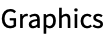Answer & check your solution
33.2Use @@ to compute the result of multiplying together integers up to 100. »
Expected output:
 Out[]=Answer & check your solution
33.3Use @@@ and Tuples to generate {f[a, a], f[a, b], f[b, a], f[b, b]}»
Expected output:
 Out[]=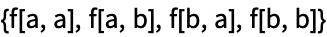Answer & check your solution
33.4Make a list of expression trees for the results of 4 successive applications of starting from x»
Expected output:
 Out[]=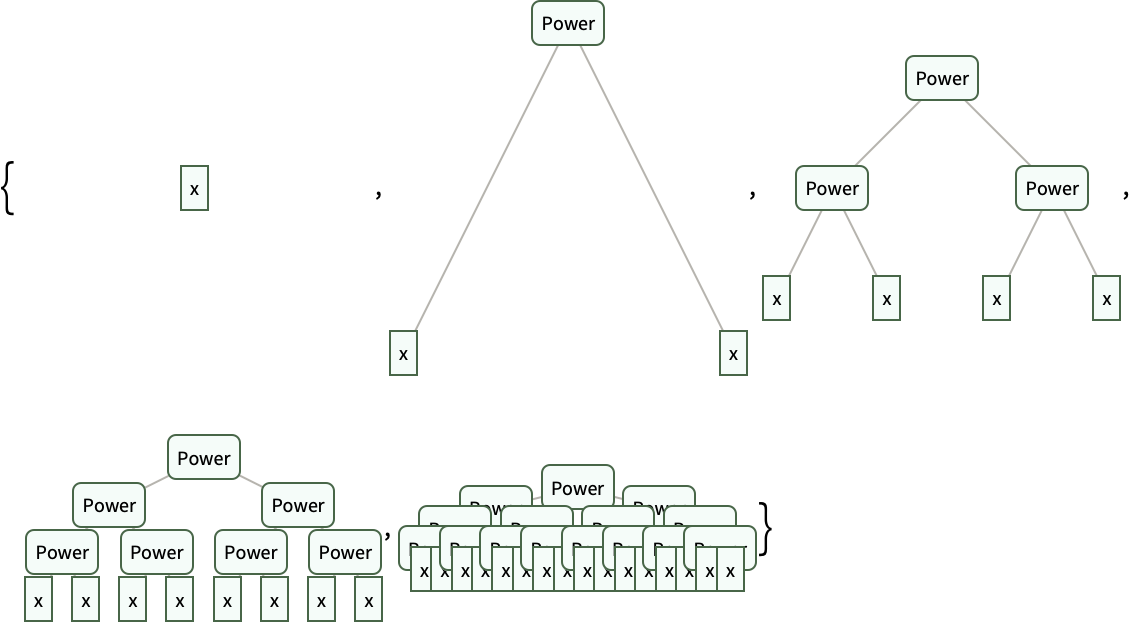Answer & check your solution
33.5Find the unique cases where i^2/(j^2+1) is an integer, with i and j going up to 20. »
Expected output:
 Out[]=Answer & check your solution
33.6Create a graph that connects successive pairs of numbers in Table[Mod[n^2+n, 100], {n, 100}]»
Expected output:
 Out[]=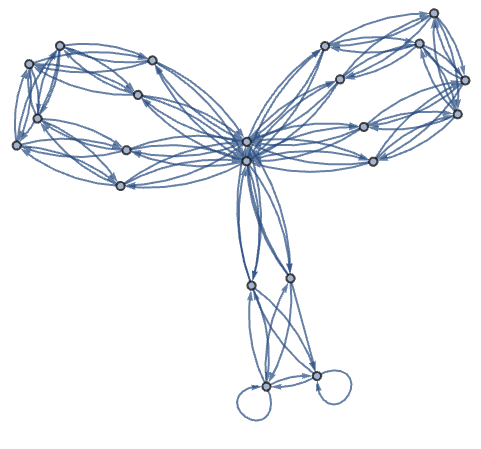Answer & check your solution
33.7Generate a graph showing which word can follow which in the first 200 words of the Wikipedia article on computers. »
Sample expected output:
 Out[]=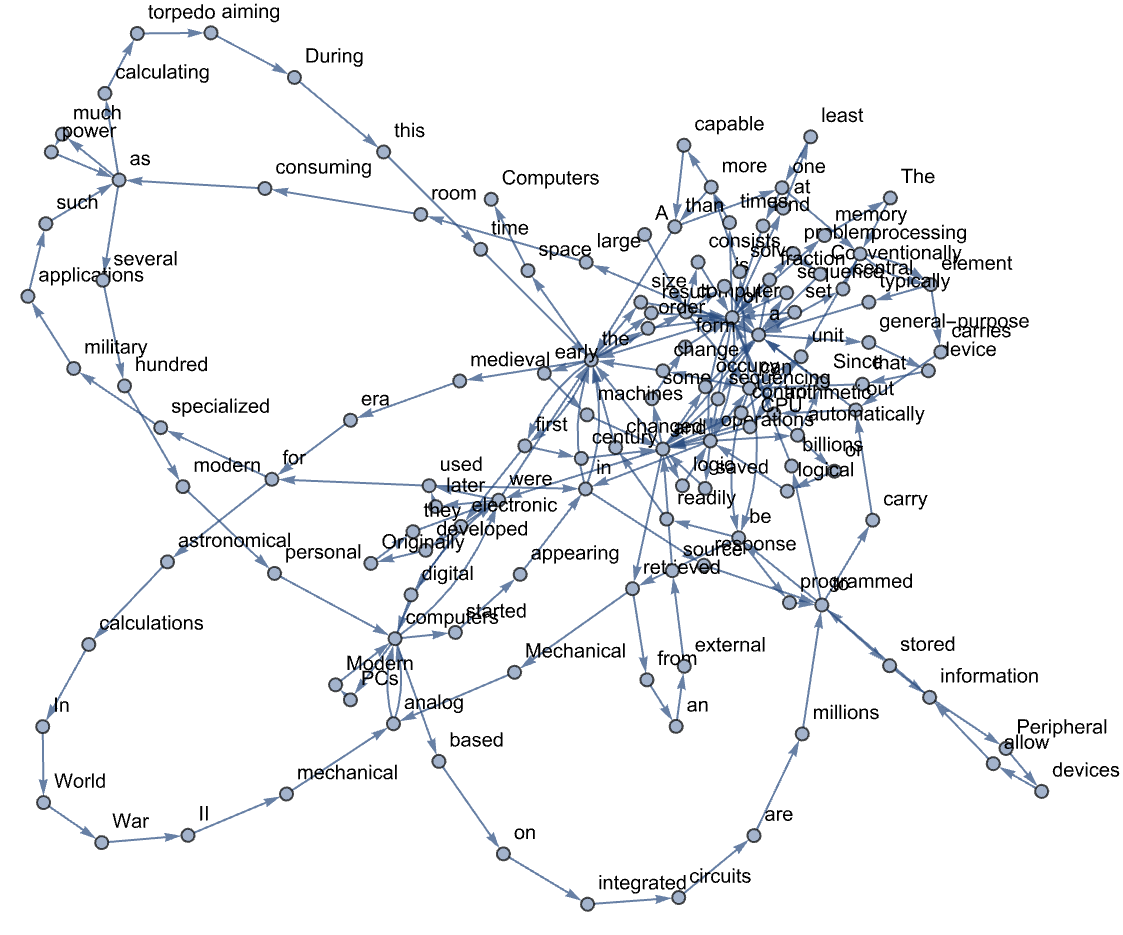Answer & check your solution
33.8Find a simpler form for f@@#&/@{{1, 2}, {7, 2}, {5, 4}}»
Sample expected output:
 Out[]=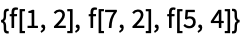Answer & check your solution
How are @@ and @@@ interpreted?
f@@expr is Apply[f, expr]. f@@@expr is MapApply[f, expr] or Apply[f, expr, {1}]. They’re usually just read as “double at” and “triple at”.
Are all expressions in the Wolfram Language trees?
At a structural level, yes. When there are variables with values assigned (see Section 38), though, they can behave more like directed graphs. And of course one can use Graph to represent any graph as an expression in the Wolfram Language.